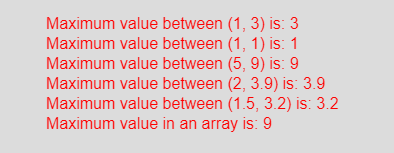Related Articles
p5.js | max() function
• Last Updated : 09 Feb, 2021

The max() function in p5.js is used to get the maximum value among sequence of numbers or two given numbers. The max() function accepts any number of parameters or an array.
Syntax:

`max(a, b)`

or

`max(arr)`

Parameters: The max(a, b) function accepts two parameters which are different number and compared to get maximum value among them. The max(arr) function accepts an array of numbers and returns the maximum value.
Return Value: It returns the maximum value between the numbers.
Below program illustrates the max() function in p5.js:
Example: This example uses max() function to get the maximum value.

## javascript

 `function` `setup() {`` ` `    ``// Create Canvas of given size``    ``createCanvas(450, 230);``}`` ` `function` `draw() {``     ` `    ``// Set the background color``    ``background(220);``     ` `    ``// Call to max() function``    ``let u = max(1, 3);``    ``let v = max(1, 1);``    ``let w = max(5, 9);``    ``let x = max(2, 3.9);``    ``let y = max(1.5, 3.2);``    ` `    ``let arr = [1, 5, 3, 8, 6, 9];``    ``let z = max(arr);``      ` `    ``// Set the size of text``    ``textSize(16);``     ` `    ``// Set the text color``    ``fill(color(``'red'``));``   ` `    ``// Getting maximum value   ``    ``text(``"Maximum value between (1, 3) is: "` `+ u, 50, 30);``    ``text(``"Maximum value between (1, 1) is: "` `+ v, 50, 50);``    ``text(``"Maximum value between (5, 9) is: "` `+ w, 50, 70);``    ``text(``"Maximum value between (2, 3.9) is: "` `+ x, 50, 90);``    ``text(``"Maximum value between (1.5, 3.2) is: "` `+ y, 50, 110);``    ``text(``"Maximum value in an array is: "` `+ z, 50, 130);``          ` `}`

Output:Reference: https://p5js.org/reference/#/p5/max

Attention reader! Don’t stop learning now. Get hold of all the important DSA concepts with the DSA Self Paced Course at a student-friendly price and become industry ready.

My Personal Notes arrow_drop_up